## What is the domain of the function f(x) =x+1/ X^2-6x+8?

Question

What is the domain of the function f(x) =x+1/
X^2-6x+8?

in progress 0
2 months 2021-07-23T21:29:30+00:00 1 Answers 3 views 0

The domain of the function is all real values of x, except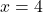and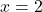Step-by-step explanation:

We are given the following function: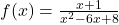It’s a fraction, so the domain is all the real values except those in which the denominator is 0.

Denominator:

Quadratic equation with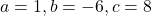Using bhaskara, the denominator is 0 for these following values of x: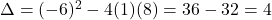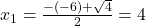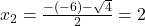The domain of the function is all real values of x, exceptand